# RS Aggarwal Solutions for Class 7 Maths Chapter 15 Properties of Triangles

RS Aggarwal Solutions for Class 7 Maths Chapter 15 – Properties of Triangles are available here. Maths is a subject which requires understanding and reasoning skills accompanied by logic. Along with this, it also requires students to practice Maths regularly. Students of Class 7 are suggested to solve RS Aggarwal Class 7 Solutions to strengthen their fundamentals and be able to solve questions that are usually asked in the examination.

The primary aim is to help students understand and crack these problems. We at BYJU’S have prepared the RS Aggarwal Solutions for Class 7 Maths Chapter 15 wherein problems are solved step by step with in-depth explanations. Download pdf of Class 7 Chapter 15 in their respective links.

## Download PDF of RS Aggarwal Solutions for Class 7 Maths Chapter 15 – Properties of Triangles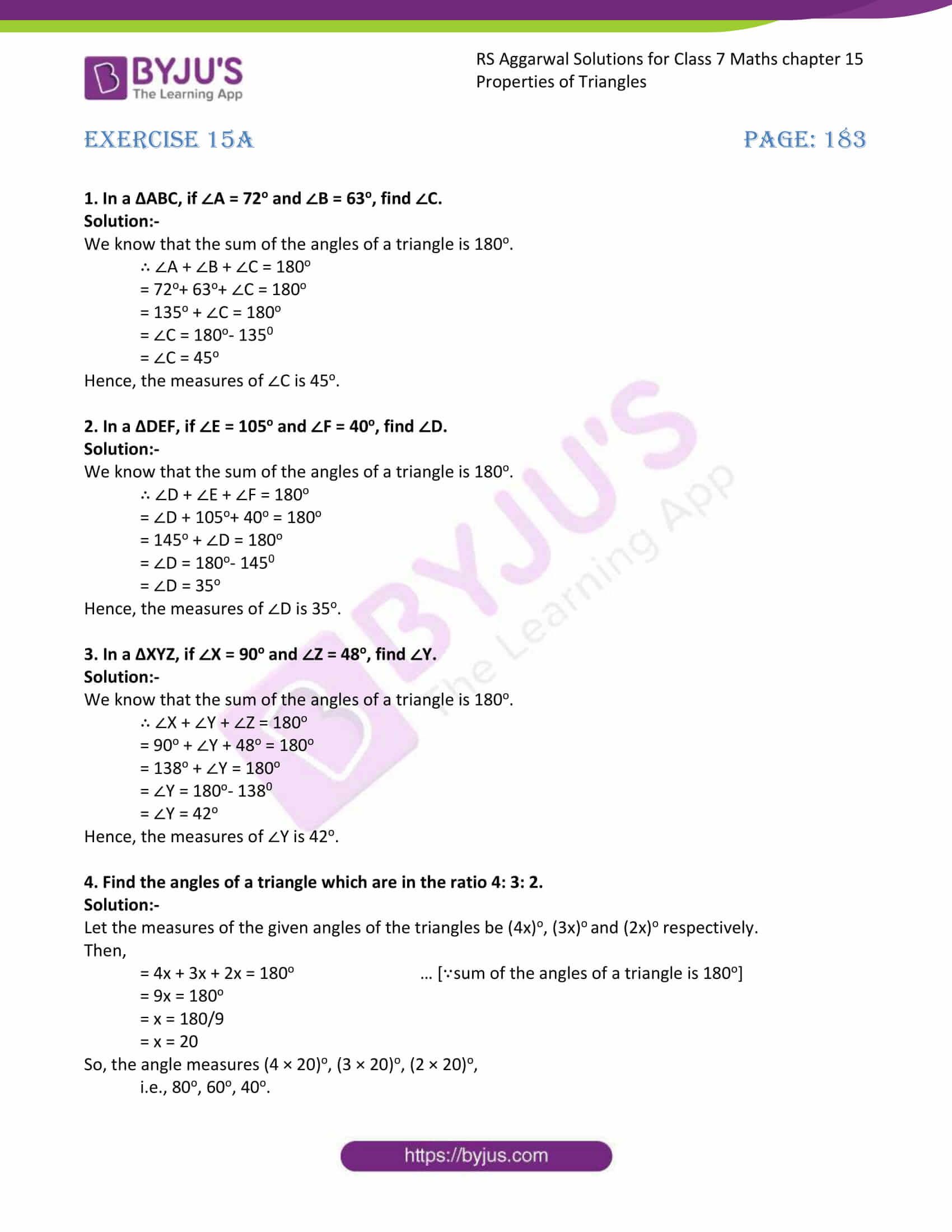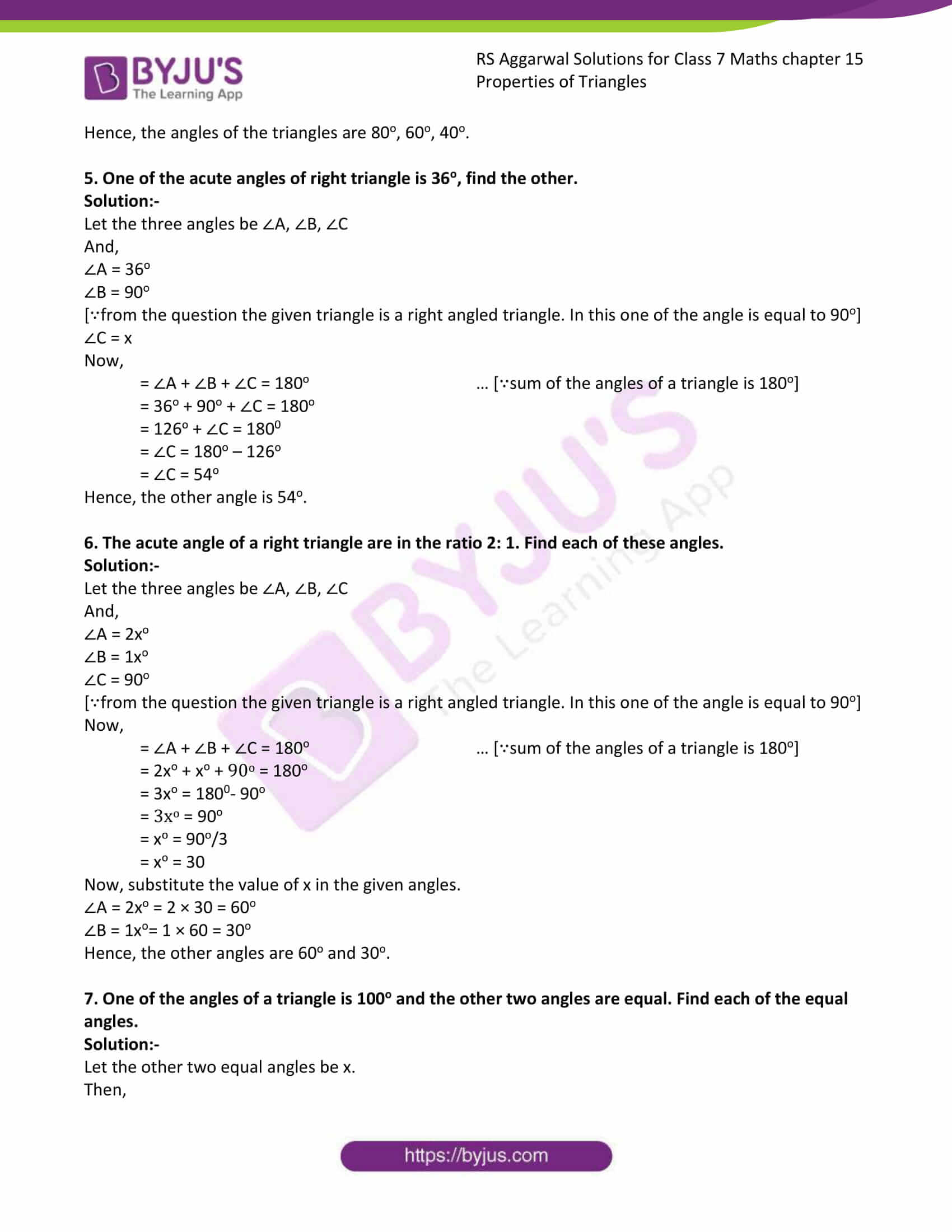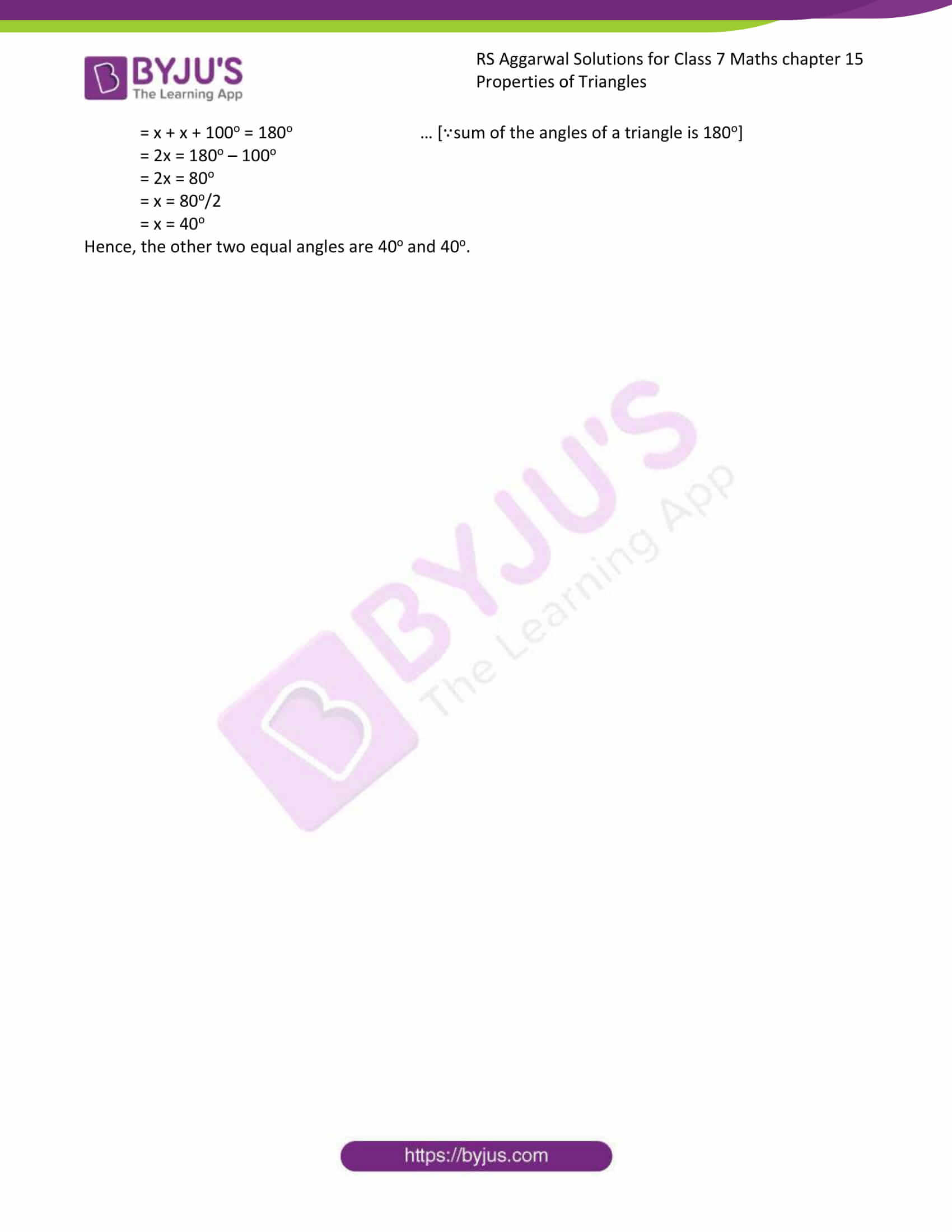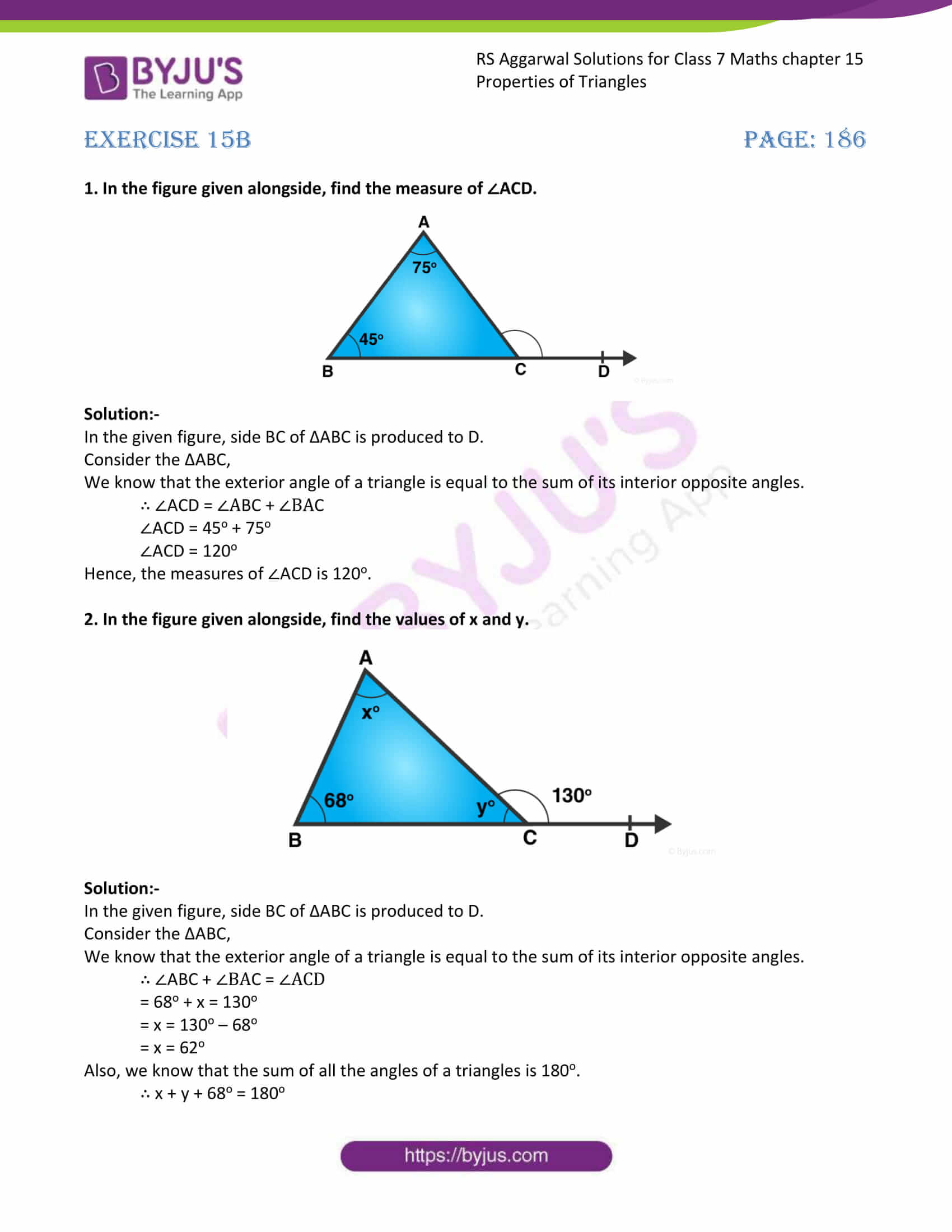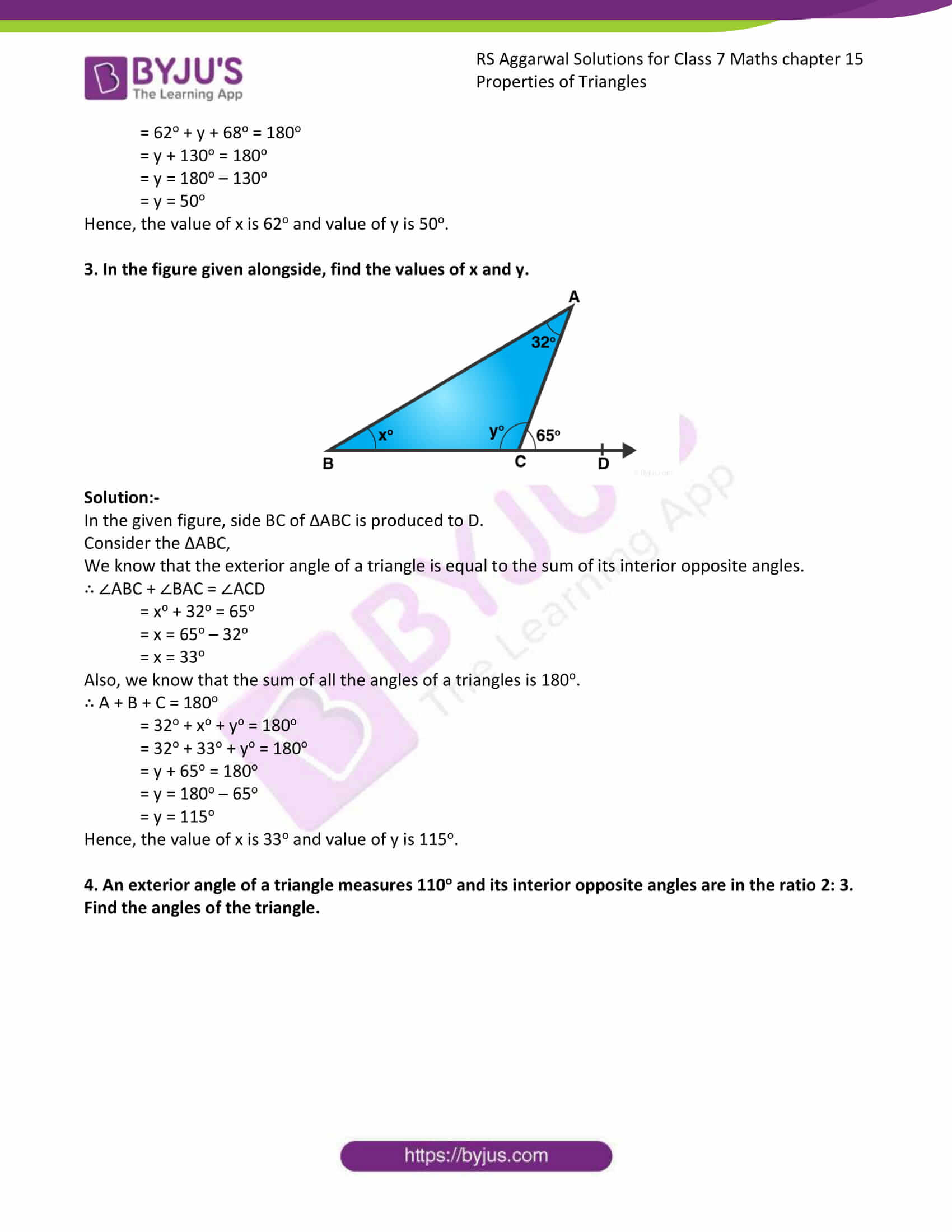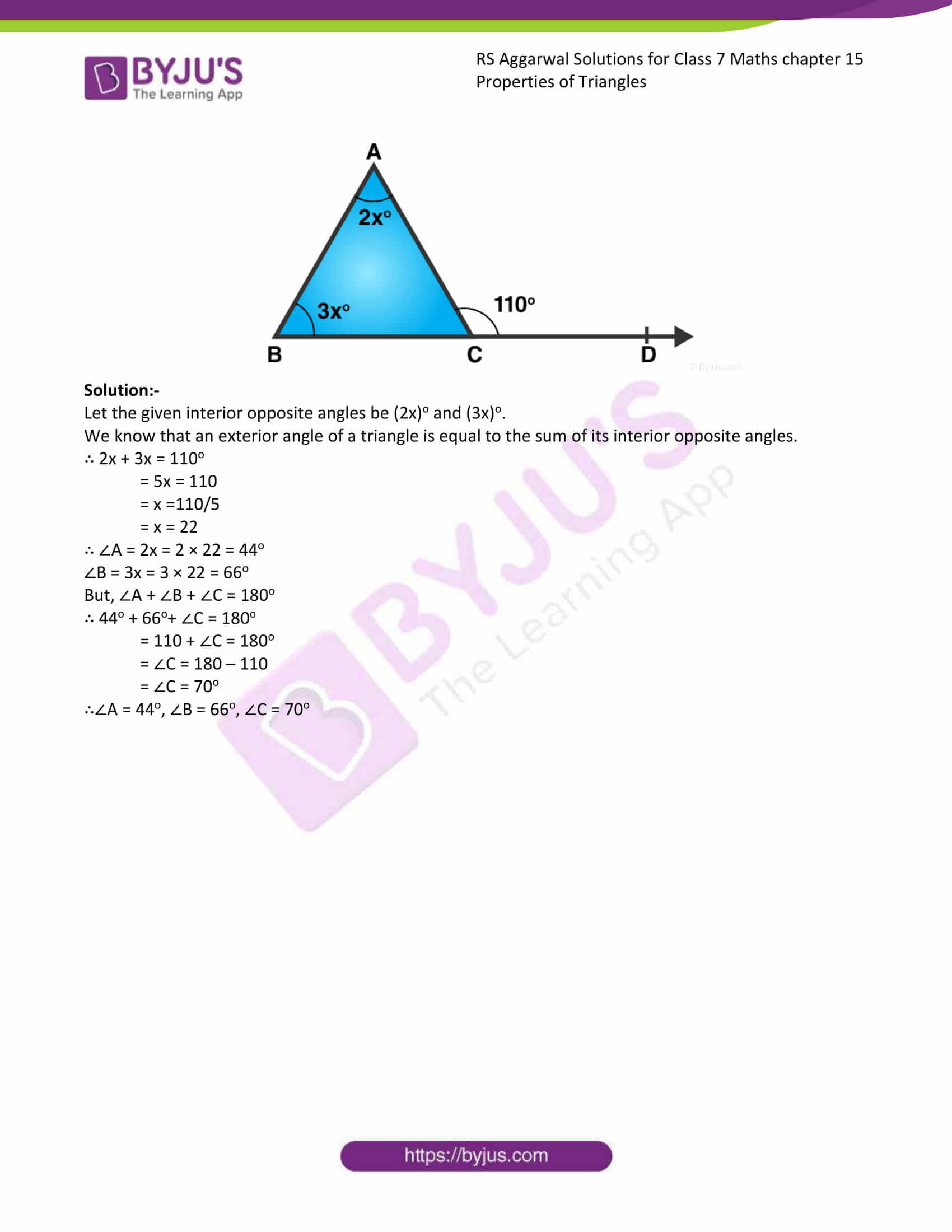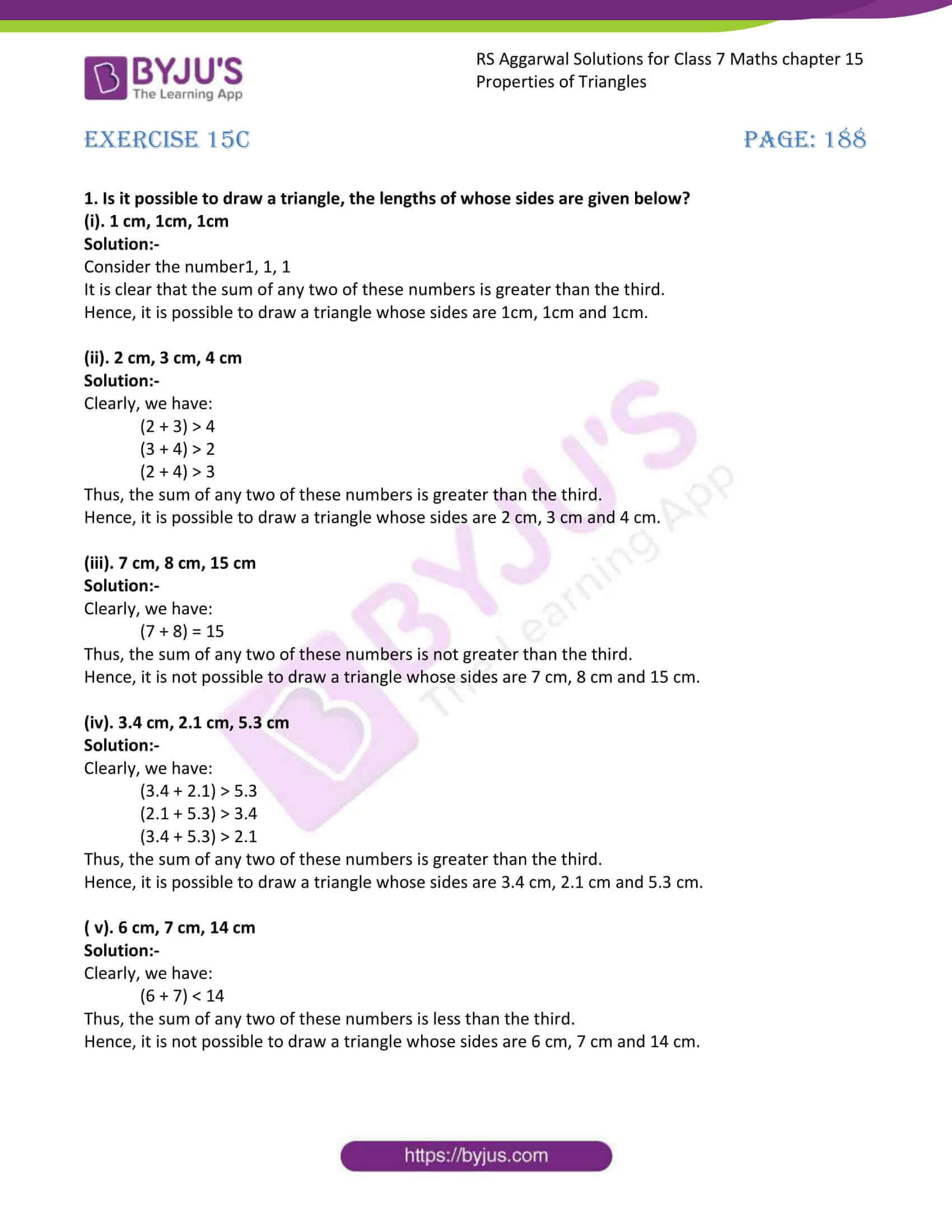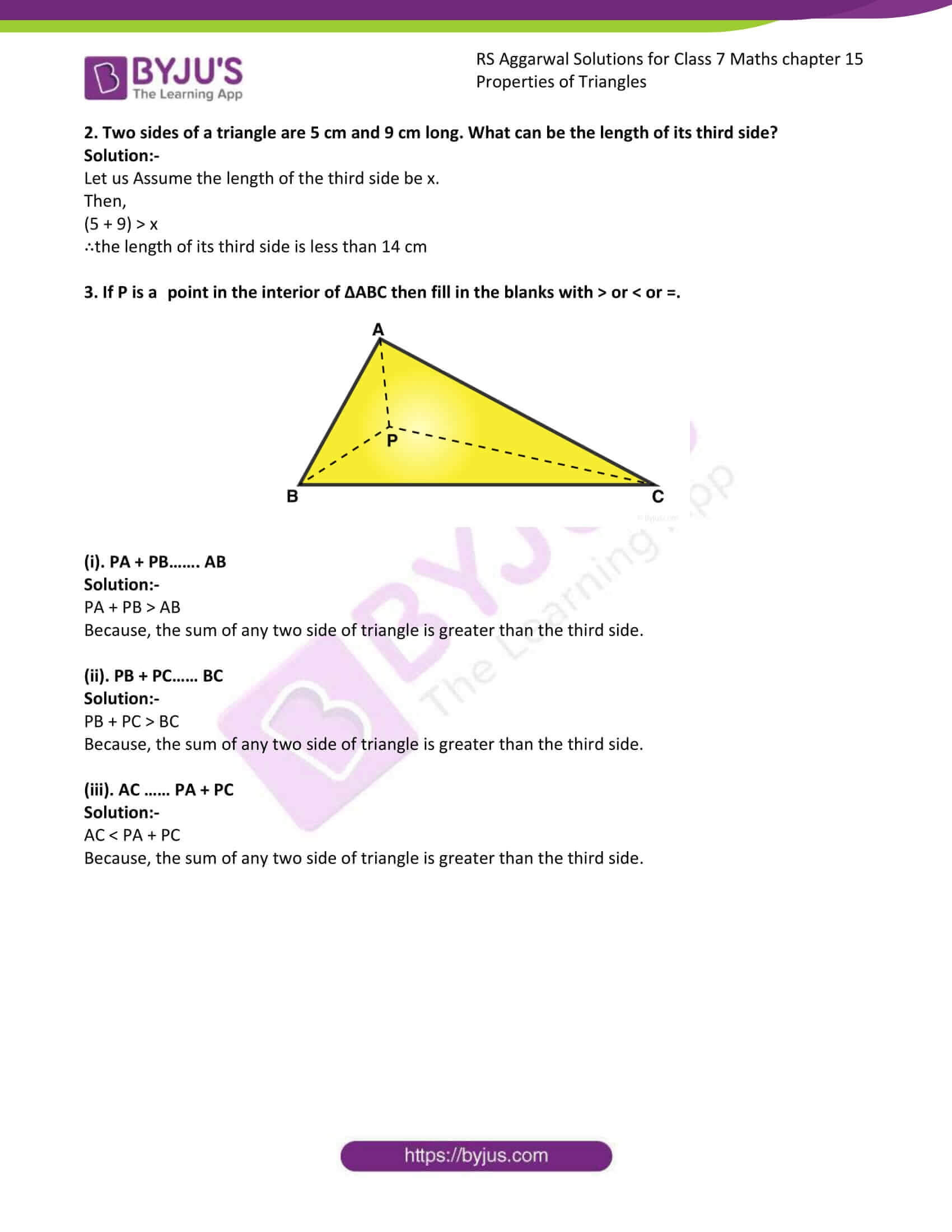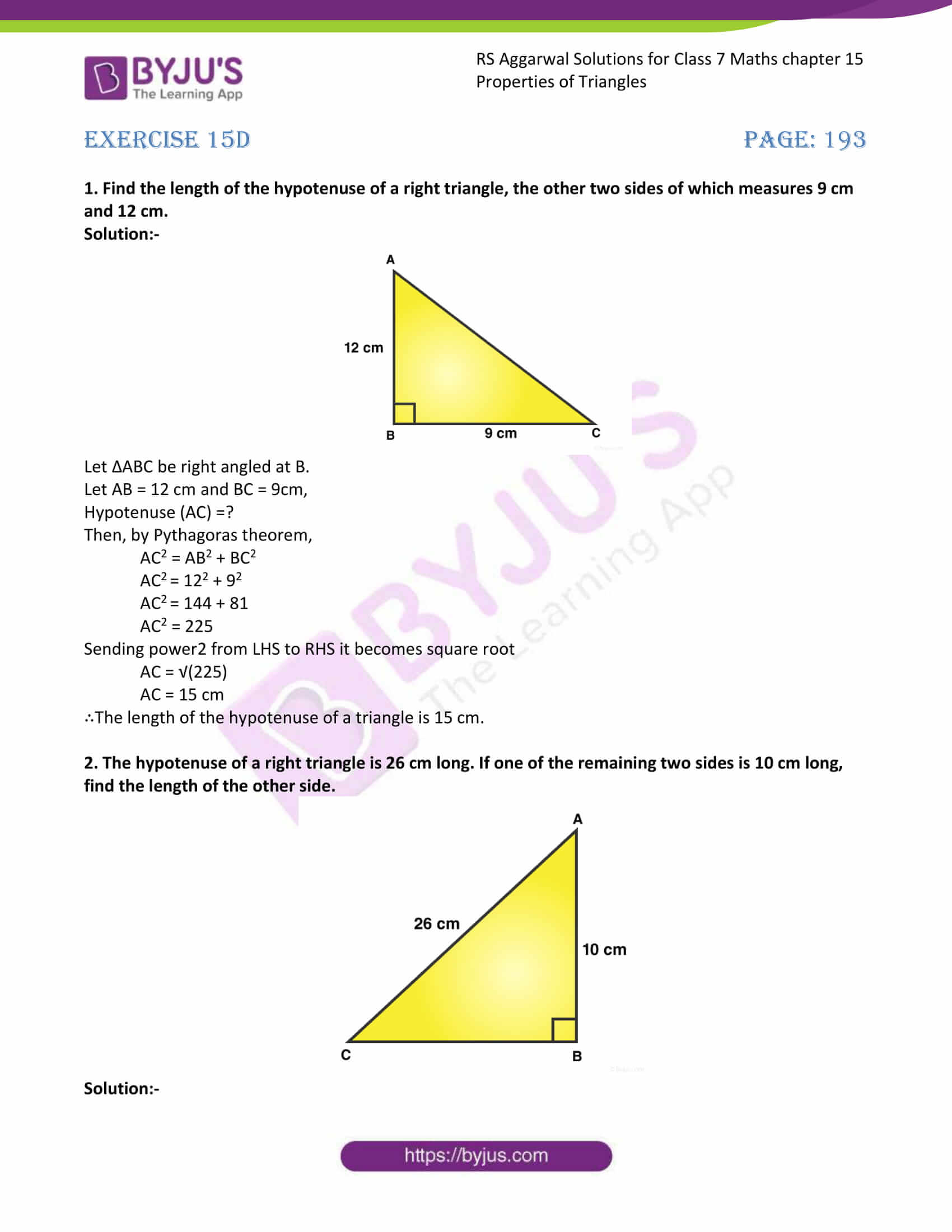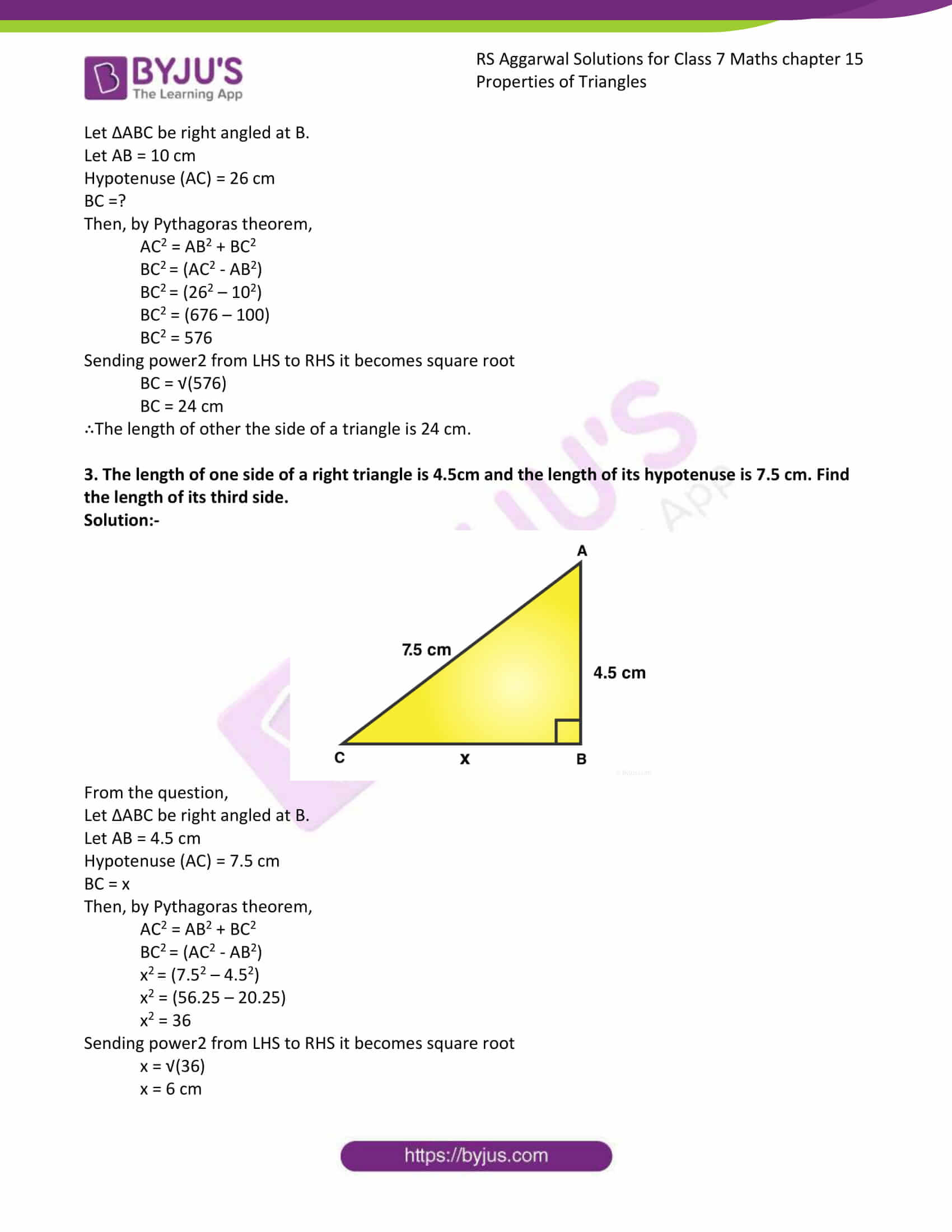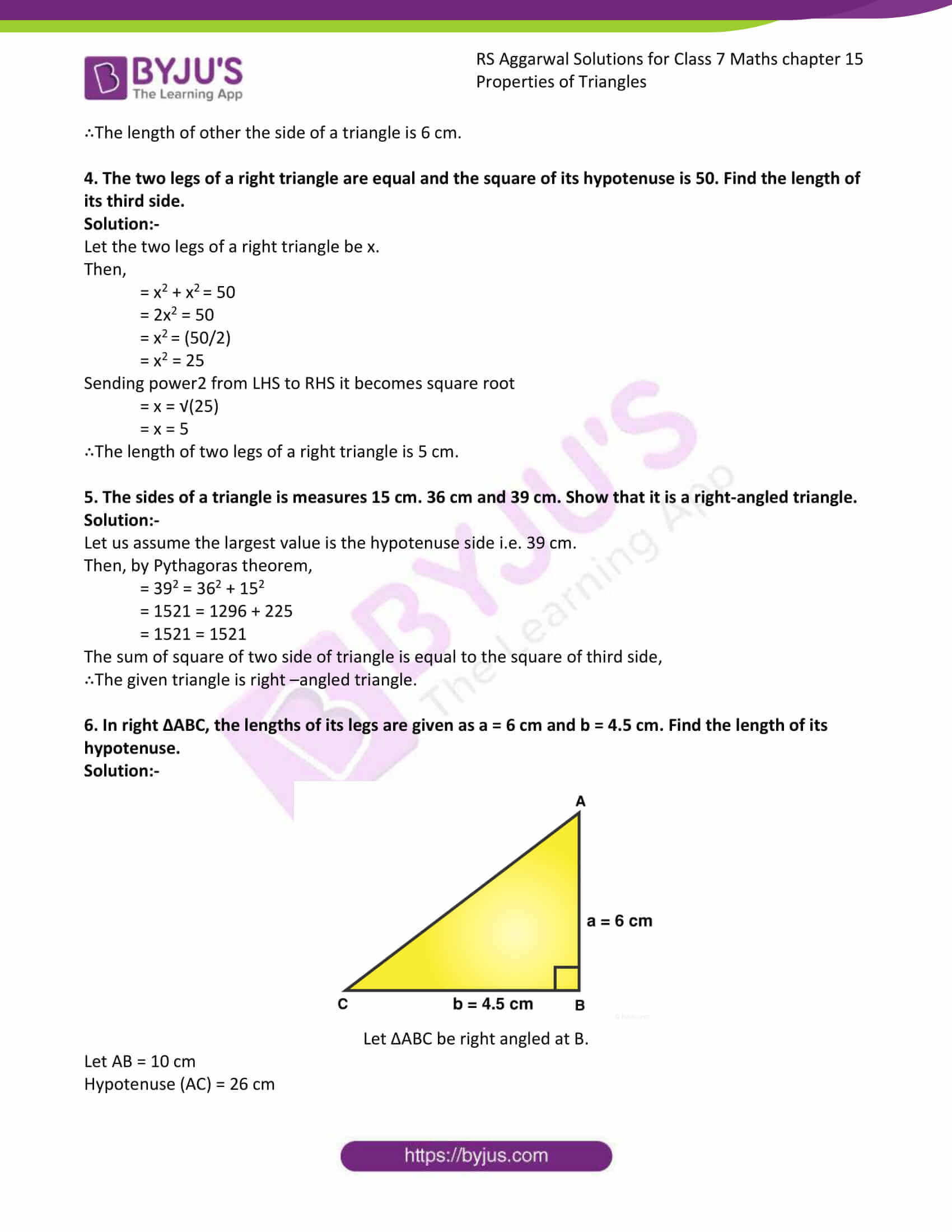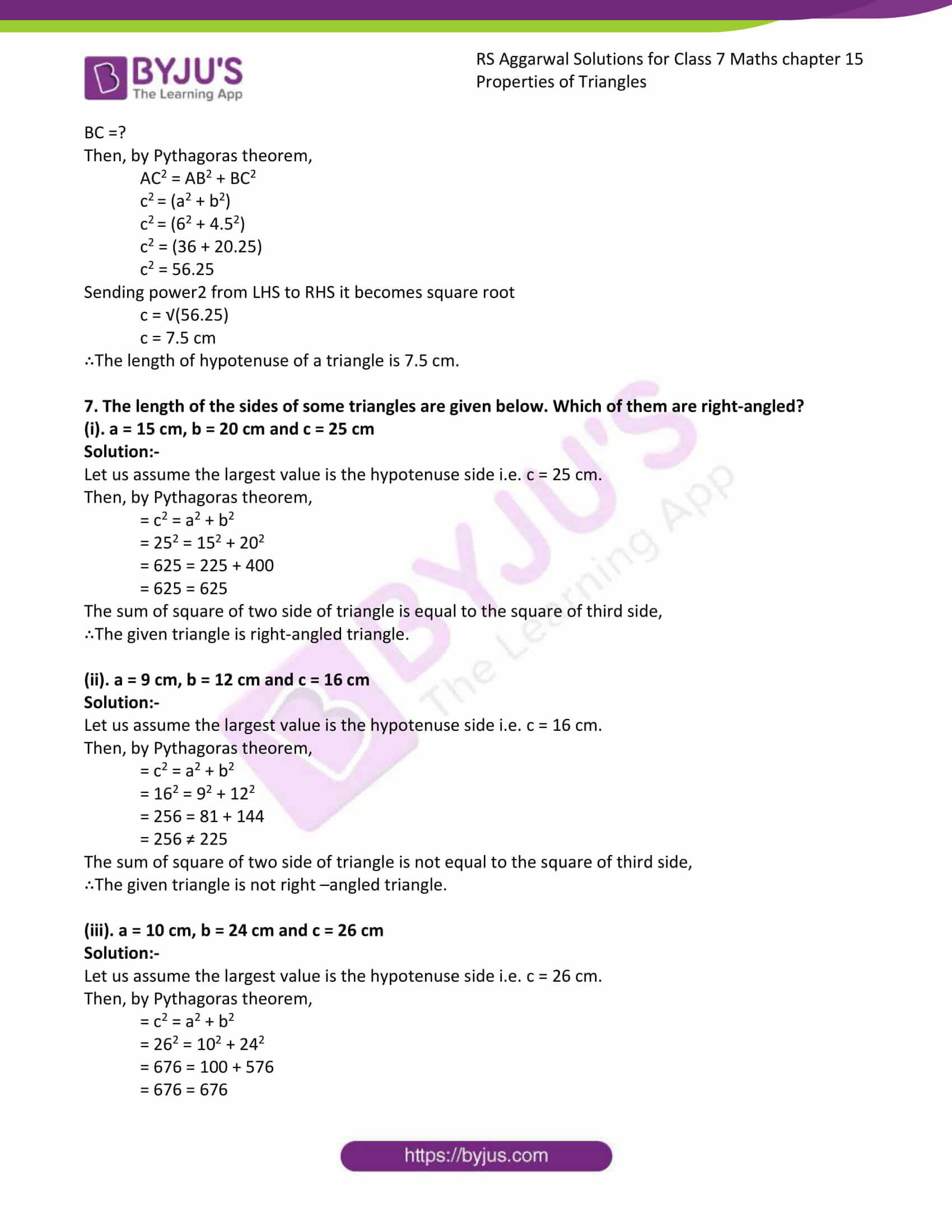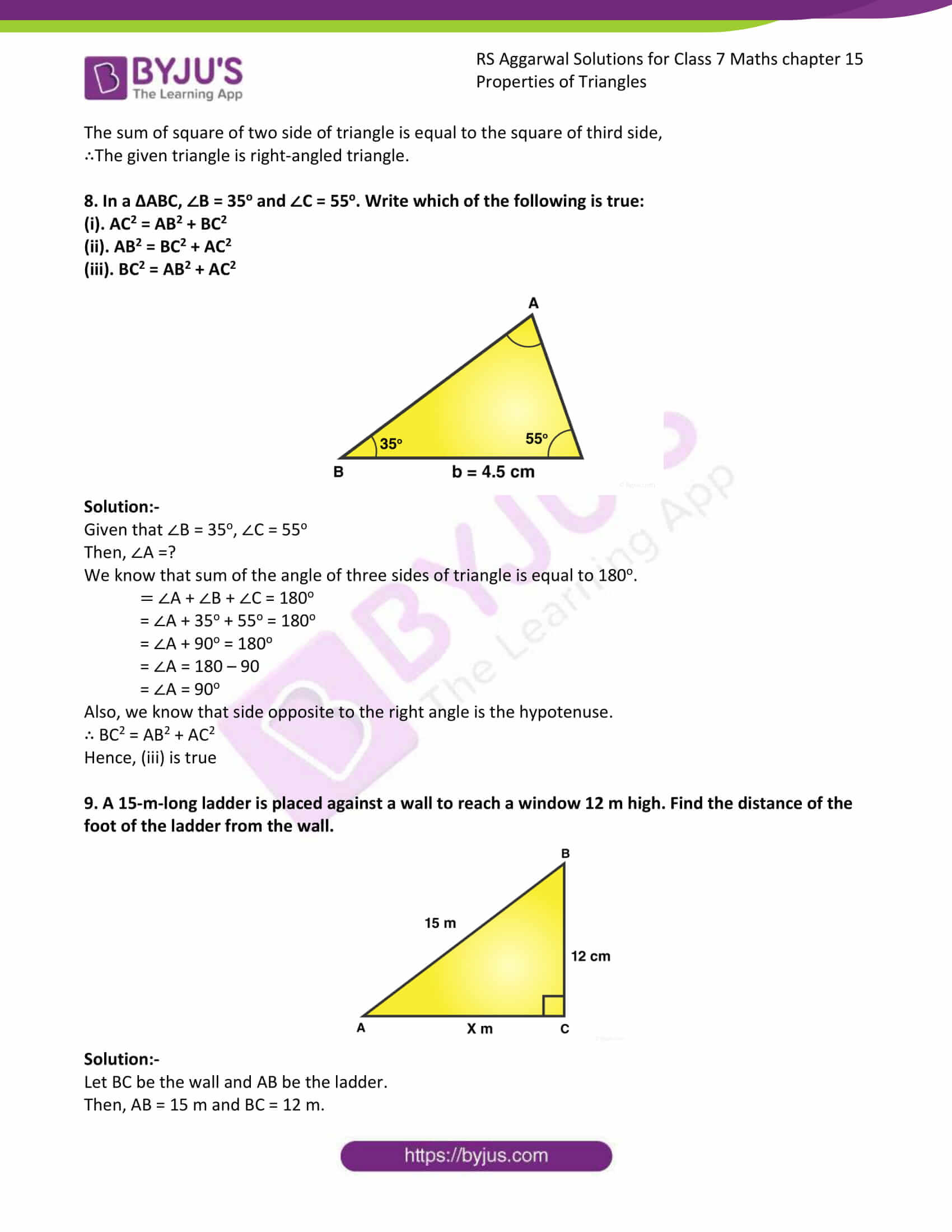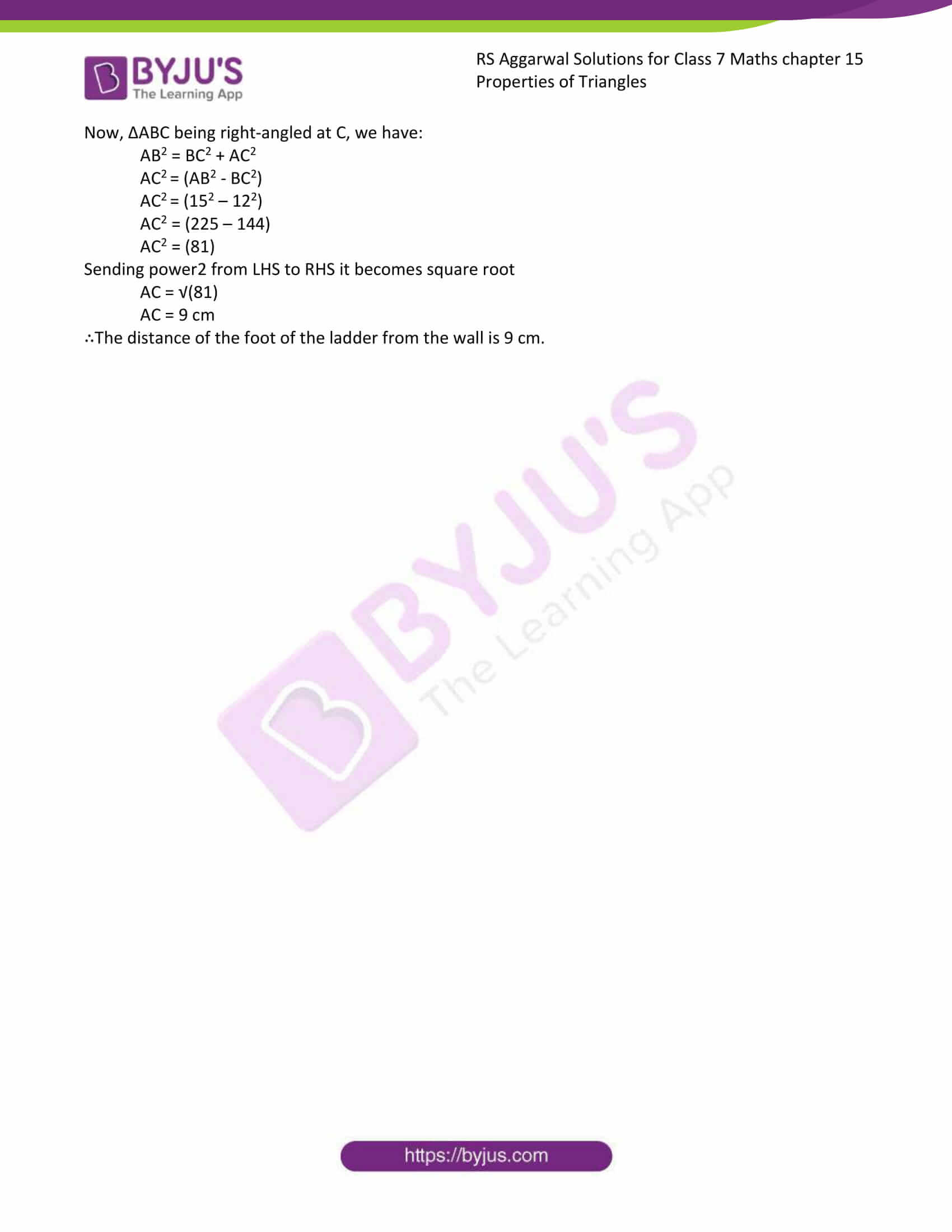Exercise 15A

Exercise 15B

Exercise 15C

Exercise 15D

## Access answers to RS Aggarwal Class 7 Maths Chapter 15 – Properties of Triangles

### Exercise 15A Page: 183

1. In a ΔABC, if ∠A = 72o and ∠B = 63o, find ∠C.

Solution:-

We know that the sum of the angles of a triangle is 180o.

∴ ∠A + ∠B + ∠C = 180o

= 72o+ 63o+ ∠C = 180o

= 135o + ∠C = 180o

= ∠C = 180o– 1350

= ∠C = 45o

Hence, the measures of ∠C is 45o.

2. In a ΔDEF, if ∠E = 105o and ∠F = 40o, find ∠D.

Solution:-

We know that the sum of the angles of a triangle is 180o.

∴ ∠D + ∠E + ∠F = 180o

= ∠D + 105o+ 40o = 180o

= 145o + ∠D = 180o

= ∠D = 180o– 1450

= ∠D = 35o

Hence, the measures of ∠D is 35o.

3. In a ΔXYZ, if ∠X = 90o and ∠Z = 48o, find ∠Y.

Solution:-

We know that the sum of the angles of a triangle is 180o.

∴ ∠X + ∠Y + ∠Z = 180o

= 90o + ∠Y + 48o = 180o

= 138o + ∠Y = 180o

= ∠Y = 180o– 1380

= ∠Y = 42o

Hence, the measures of ∠Y is 42o.

4. Find the angles of a triangle which are in the ratio 4: 3: 2.

Solution:-

Let the measures of the given angles of the triangles be (4x)o, (3x)o and (2x)o respectively.

Then,

= 4x + 3x + 2x = 180o … [∵sum of the angles of a triangle is 180o]

= 9x = 180o

= x = 180/9

= x = 20

So, the angle measures (4 × 20)o, (3 × 20)o, (2 × 20)o,

i.e., 80o, 60o, 40o.

Hence, the angles of the triangles are 80o, 60o, 40o.

5. One of the acute angles of right triangle is 36o, find the other.

Solution:-

Let the three angles be ∠A, ∠B, ∠C

And,

∠A = 36o

∠B = 90o

[∵from the question the given triangle is a right angled triangle. In this one of the angle is equal to 90o]

∠C = x

Now,

= ∠A + ∠B + ∠C = 180o … [∵sum of the angles of a triangle is 180o]

= 36o + 90o + ∠C = 180o

= 126o + ∠C = 1800

= ∠C = 180o – 126o

= ∠C = 54o

Hence, the other angle is 54o.

6. The acute angle of a right triangle are in the ratio 2: 1. Find each of these angles.

Solution:-

Let the three angles be ∠A, ∠B, ∠C

And,

∠A = 2xo

∠B = 1xo

∠C = 90o

[∵from the question the given triangle is a right angled triangle. In this one of the angle is equal to 90o]

Now,

= ∠A + ∠B + ∠C = 180o … [∵sum of the angles of a triangle is 180o]

= 2xo + xo + 90o = 180o

= 3xo = 1800– 90o

= 3xo = 90o

= xo = 90o/3

= xo = 30

Now, substitute the value of x in the given angles.

∠A = 2xo = 2 × 30 = 60o

∠B = 1xo= 1 × 60 = 30o

Hence, the other angles are 60o and 30o.

7. One of the angles of a triangle is 100o and the other two angles are equal. Find each of the equal angles.

Solution:-

Let the other two equal angles be x.

Then,

= x + x + 100o = 180o … [∵sum of the angles of a triangle is 180o]

= 2x = 180o – 100o

= 2x = 80o

= x = 80o/2

= x = 40o

Hence, the other two equal angles are 40o and 40o.

Exercise 15B Page: 186

1. In the figure given alongside, find the measure of ∠ACD.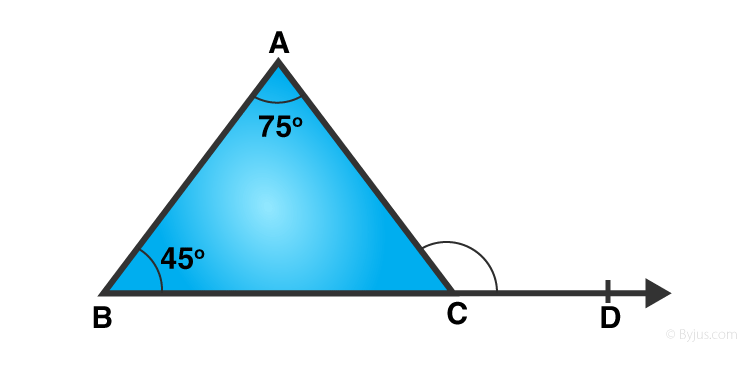Solution:-

In the given figure, side BC of ΔABC is produced to D.

Consider the ΔABC,

We know that the exterior angle of a triangle is equal to the sum of its interior opposite angles.

∴ ∠ACD = ∠ABC + ∠BAC

∠ACD = 45o + 75o

∠ACD = 120o

Hence, the measures of ∠ACD is 120o.

2. In the figure given alongside, find the values of x and y.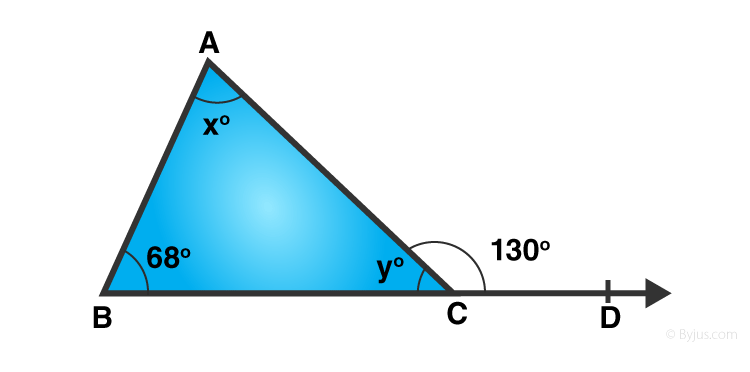Solution:-

In the given figure, side BC of ΔABC is produced to D.

Consider the ΔABC,

We know that the exterior angle of a triangle is equal to the sum of its interior opposite angles.

∴ ∠ABC + ∠BAC = ∠ACD

= 68o + x = 130o

= x = 130o – 68o

= x = 62o

Also, we know that the sum of all the angles of a triangles is 180o.

∴ x + y + 68o = 180o

= 62o + y + 68o = 180o

= y + 130o = 180o

= y = 180o – 130o

= y = 50o

Hence, the value of x is 62o and value of y is 50o.

3. In the figure given alongside, find the values of x and y.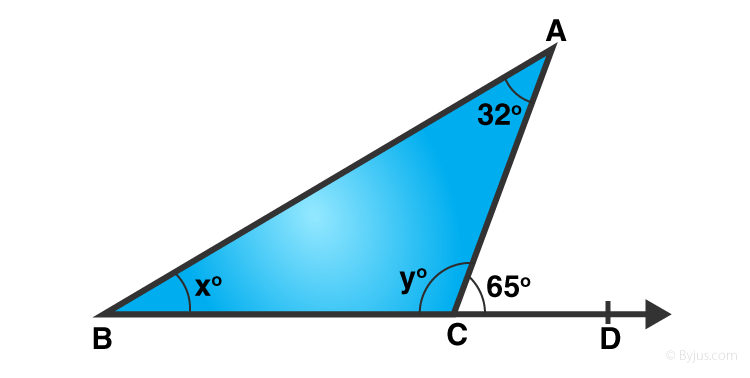Solution:-

In the given figure, side BC of ΔABC is produced to D.

Consider the ΔABC,

We know that the exterior angle of a triangle is equal to the sum of its interior opposite angles.

∴ ∠ABC + ∠BAC = ∠ACD

= xo + 32o = 65o

= x = 65o – 32o

= x = 33o

Also, we know that the sum of all the angles of a triangles is 180o.

∴ A + B + C = 180o

= 32o + xo + yo = 180o

= 32o + 33o + yo = 180o

= y + 65o = 180o

= y = 180o – 65o

= y = 115o

Hence, the value of x is 33o and value of y is 115o.

4. An exterior angle of a triangle measures 110o and its interior opposite angles are in the ratio 2: 3. Find the angles of the triangle.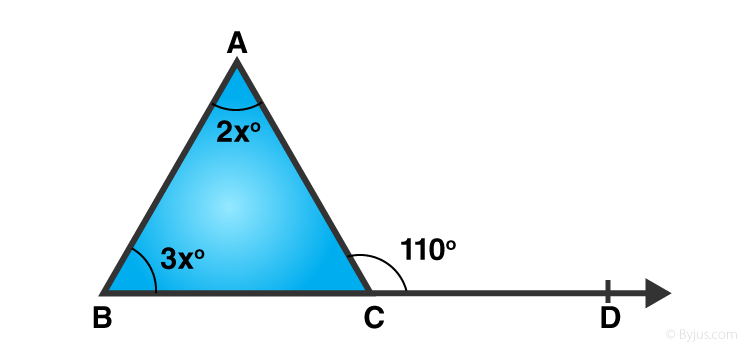Solution:-

Let the given interior opposite angles be (2x)o and (3x)o.

We know that an exterior angle of a triangle is equal to the sum of its interior opposite angles.

∴ 2x + 3x = 110o

= 5x = 110

= x =110/5

= x = 22

∴ ∠A = 2x = 2 × 22 = 44o

∠B = 3x = 3 × 22 = 66o

But, ∠A + ∠B + ∠C = 180o

∴ 44o + 66o+ ∠C = 180o

= 110 + ∠C = 180o

= ∠C = 180 – 110

= ∠C = 70o

∴∠A = 44o, ∠B = 66o, ∠C = 70o

Exercise 15C Page: 188

1. Is it possible to draw a triangle, the lengths of whose sides are given below?

(i). 1 cm, 1cm, 1cm

Solution:-

Consider the number1, 1, 1

It is clear that the sum of any two of these numbers is greater than the third.

Hence, it is possible to draw a triangle whose sides are 1cm, 1cm and 1cm.

(ii). 2 cm, 3 cm, 4 cm

Solution:-

Clearly, we have:

(2 + 3) > 4

(3 + 4) > 2

(2 + 4) > 3

Thus, the sum of any two of these numbers is greater than the third.

Hence, it is possible to draw a triangle whose sides are 2 cm, 3 cm and 4 cm.

(iii). 7 cm, 8 cm, 15 cm

Solution:-

Clearly, we have:

(7 + 8) = 15

Thus, the sum of any two of these numbers is not greater than the third.

Hence, it is not possible to draw a triangle whose sides are 7 cm, 8 cm and 15 cm.

(iv). 3.4 cm, 2.1 cm, 5.3 cm

Solution:-

Clearly, we have:

(3.4 + 2.1) > 5.3

(2.1 + 5.3) > 3.4

(3.4 + 5.3) > 2.1

Thus, the sum of any two of these numbers is greater than the third.

Hence, it is possible to draw a triangle whose sides are 3.4 cm, 2.1 cm and 5.3 cm.

(v). 6 cm, 7 cm, 14 cm

Solution:-

Clearly, we have:

(6 + 7) < 14

Thus, the sum of any two of these numbers is less than the third.

Hence, it is not possible to draw a triangle whose sides are 6 cm, 7 cm and 14 cm.

2. Two sides of a triangle are 5 cm and 9 cm long. What can be the length of its third side?

Solution:-

Let us Assume the length of the third side be x.

Then,

(5 + 9) > x

∴the length of its third side is less than 14 cm

3. If P is a point in the interior of ΔABC then fill in the blanks with > or < or =.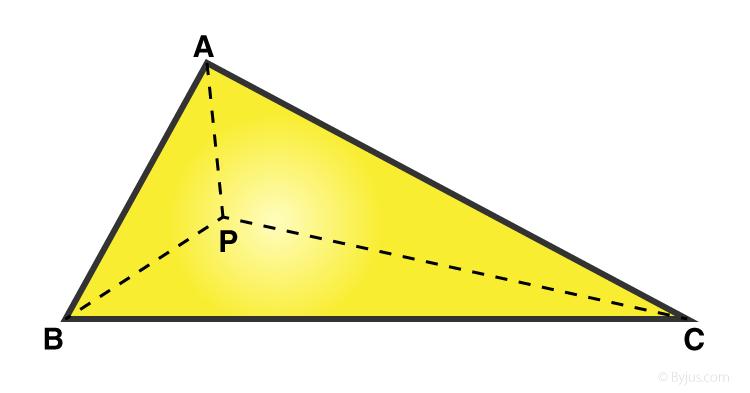(i). PA + PB……. AB

Solution:-

PA + PB > AB

Because, the sum of any two side of triangle is greater than the third side.

(ii). PB + PC…… BC

Solution:-

PB + PC > BC

Because, the sum of any two side of triangle is greater than the third side.

(iii). AC …… PA + PC

Solution:-

AC < PA + PC

Because, the sum of any two side of triangle is greater than the third side.

Exercise 15D Page: 193

1. Find the length of the hypotenuse of a right triangle, the other two sides of which measures 9 cm and 12 cm.

Solution:-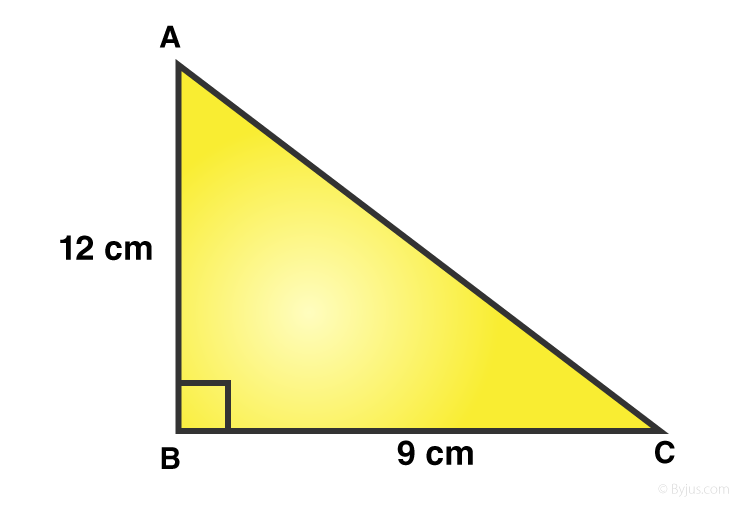Let ΔABC be right angled at B.

Let AB = 12 cm and BC = 9cm,

Hypotenuse (AC) =?

Then, by Pythagoras theorem,

AC2 = AB2 + BC2

AC2 = 122 + 92

AC2 = 144 + 81

AC2 = 225

Sending power2 from LHS to RHS it becomes square root

AC = √(225)

AC = 15 cm

∴The length of the hypotenuse of a triangle is 15 cm.

2. The hypotenuse of a right triangle is 26 cm long. If one of the remaining two sides is 10 cm long, find the length of the other side.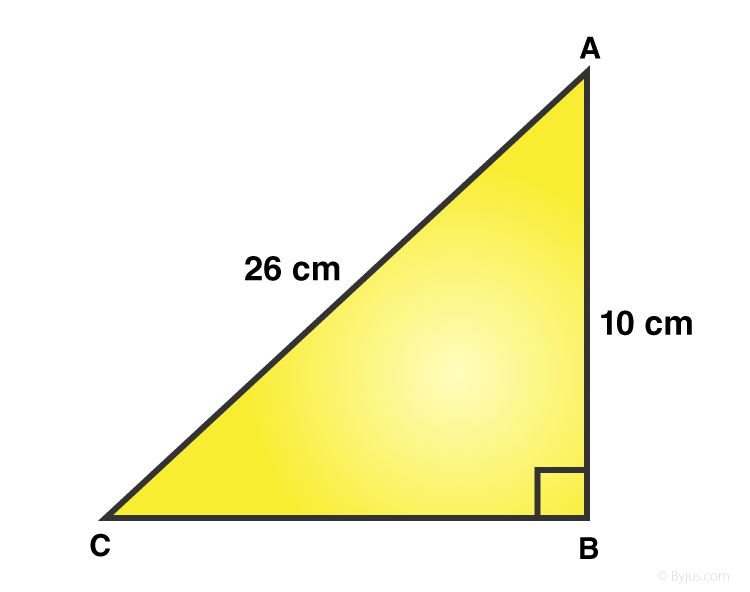Solution:-

Let ΔABC be right angled at B.

Let AB = 10 cm

Hypotenuse (AC) = 26 cm

BC =?

Then, by Pythagoras theorem,

AC2 = AB2 + BC2

BC2 = (AC2 – AB2)

BC2 = (262 – 102)

BC2 = (676 – 100)

BC2 = 576

Sending power2 from LHS to RHS it becomes square root

BC = √(576)

BC = 24 cm

∴The length of other the side of a triangle is 24 cm.

3. The length of one side of a right triangle is 4.5cm and the length of its hypotenuse is 7.5 cm. Find the length of its third side.

Solution:-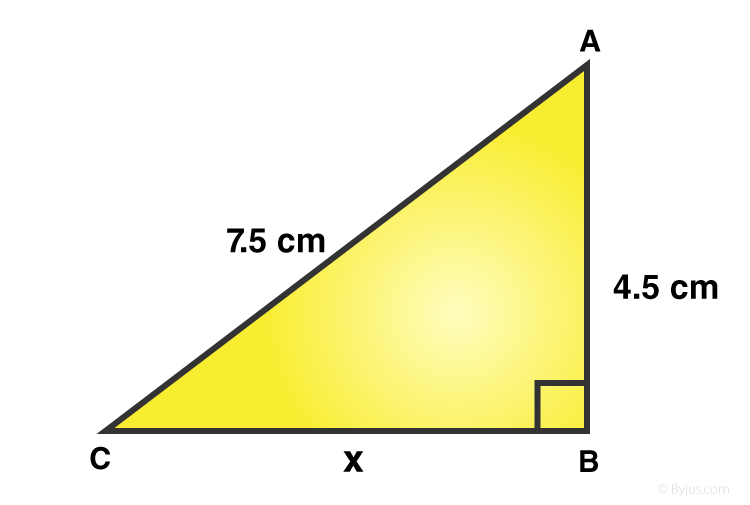From the question,

Let ΔABC be right angled at B.

Let AB = 4.5 cm

Hypotenuse (AC) = 7.5 cm

BC = x

Then, by Pythagoras theorem,

AC2 = AB2 + BC2

BC2 = (AC2 – AB2)

x2 = (7.52 – 4.52)

x2 = (56.25 – 20.25)

x2 = 36

Sending power2 from LHS to RHS it becomes square root

x = √(36)

x = 6 cm

∴The length of other the side of a triangle is 6 cm.

4. The two legs of a right triangle are equal and the square of its hypotenuse is 50. Find the length of its third side.

Solution:-

Let the two legs of a right triangle be x.

Then,

= x2 + x2 = 50

= 2x2 = 50

= x2 = (50/2)

= x2 = 25

Sending power2 from LHS to RHS it becomes square root

= x = √(25)

= x = 5

∴The length of two legs of a right triangle is 5 cm.

5. The sides of a triangle is measures 15 cm. 36 cm and 39 cm. Show that it is a right-angled triangle.

Solution:-

Let us assume the largest value is the hypotenuse side i.e. 39 cm.

Then, by Pythagoras theorem,

= 392 = 362 + 152

= 1521 = 1296 + 225

= 1521 = 1521

The sum of square of two side of triangle is equal to the square of third side,

∴The given triangle is right –angled triangle.

6. In right ΔABC, the lengths of its legs are given as a = 6 cm and b = 4.5 cm. Find the length of its hypotenuse.

Solution:-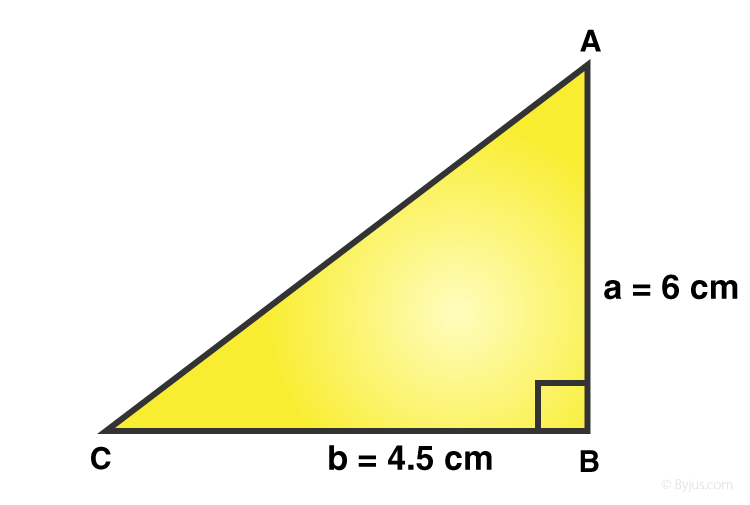Let ΔABC be right angled at B.

Let AB = 10 cm

Hypotenuse (AC) = 26 cm

BC =?

Then, by Pythagoras theorem,

AC2 = AB2 + BC2

c2 = (a2 + b2)

c2 = (62 + 4.52)

c2 = (36 + 20.25)

c2 = 56.25

Sending power2 from LHS to RHS it becomes square root

c = √(56.25)

c = 7.5 cm

∴The length of hypotenuse of a triangle is 7.5 cm.

7. The length of the sides of some triangles are given below. Which of them are right-angled?

(i). a = 15 cm, b = 20 cm and c = 25 cm

Solution:-

Let us assume the largest value is the hypotenuse side i.e. c = 25 cm.

Then, by Pythagoras theorem,

= c2 = a2 + b2

= 252 = 152 + 202

= 625 = 225 + 400

= 625 = 625

The sum of square of two side of triangle is equal to the square of third side,

∴The given triangle is right-angled triangle.

(ii). a = 9 cm, b = 12 cm and c = 16 cm

Solution:-

Let us assume the largest value is the hypotenuse side i.e. c = 16 cm.

Then, by Pythagoras theorem,

= c2 = a2 + b2

= 162 = 92 + 122

= 256 = 81 + 144

= 256 ≠ 225

The sum of square of two side of triangle is not equal to the square of third side,

∴The given triangle is not right –angled triangle.

(iii). a = 10 cm, b = 24 cm and c = 26 cm

Solution:-

Let us assume the largest value is the hypotenuse side i.e. c = 26 cm.

Then, by Pythagoras theorem,

= c2 = a2 + b2

= 262 = 102 + 242

= 676 = 100 + 576

= 676 = 676

The sum of square of two side of triangle is equal to the square of third side,

∴The given triangle is right-angled triangle.

8. In a ΔABC, ∠B = 35o and ∠C = 55o. Write which of the following is true:

(i). AC2 = AB2 + BC2

(ii). AB2 = BC2 + AC2

(iii). BC2 = AB2 + AC2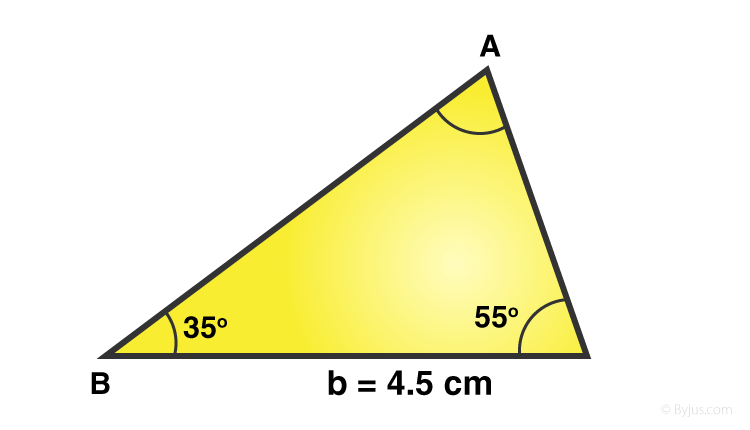Solution:-

Given that ∠B = 35o, ∠C = 55o

Then, ∠A =?

We know that sum of the angle of three sides of triangle is equal to 180o.

= ∠A + ∠B + ∠C = 180o

= ∠A + 35o + 55o = 180o

= ∠A + 90o = 180o

= ∠A = 180 – 90

= ∠A = 90o

Also, we know that side opposite to the right angle is the hypotenuse.

∴ BC2 = AB2 + AC2

Hence, (iii) is true

9. A 15-m-long ladder is placed against a wall to reach a window 12 m high. Find the distance of the foot of the ladder from the wall.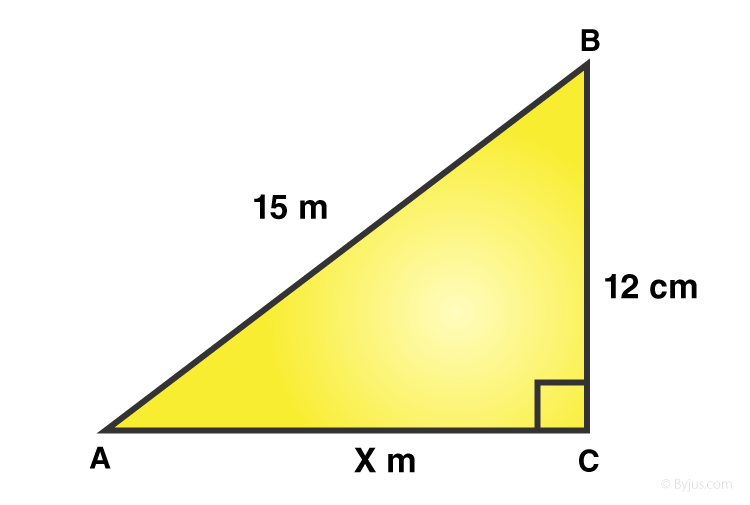Solution:-

Let BC be the wall and AB be the ladder.

Then, AB = 15 m and BC = 12 m.

Now, ΔABC being right-angled at C, we have:

AB2 = BC2 + AC2

AC2 = (AB2 – BC2)

AC2 = (152 – 122)

AC2 = (225 – 144)

AC2 = (81)

Sending power2 from LHS to RHS it becomes square root

AC = √(81)

AC = 9 cm

∴The distance of the foot of the ladder from the wall is 9 cm.

## RS Aggarwal Solutions for Class 7 Maths Chapter 15 – Properties of Triangles

Chapter 15 – Properties of Triangles contains 4 exercises and the RS Aggarwal Solutions available on this page provide solutions to the questions present in the exercises. Now, let us have a look at some of the concepts discussed in this chapter.

• Various Types of Triangles
• Naming Triangles by Considering their Angles
• Angle Sum Property of a Triangle
• Exterior and Interior Opposite Angles
• Exterior Angles Property of a Triangle
• Triangle Inequality
• Pythagoras Theorem

### Chapter Brief of RS Aggarwal Solutions for Class 7 Maths Chapter 15 – Properties of Triangles

RS Aggarwal Solutions for Class 7 Maths Chapter 15 – Properties of Triangles. In Class 6, students learnt the definition of a triangle and various types of triangles based on angles and sides. Now, in this chapter review these results and study some more properties of triangles.